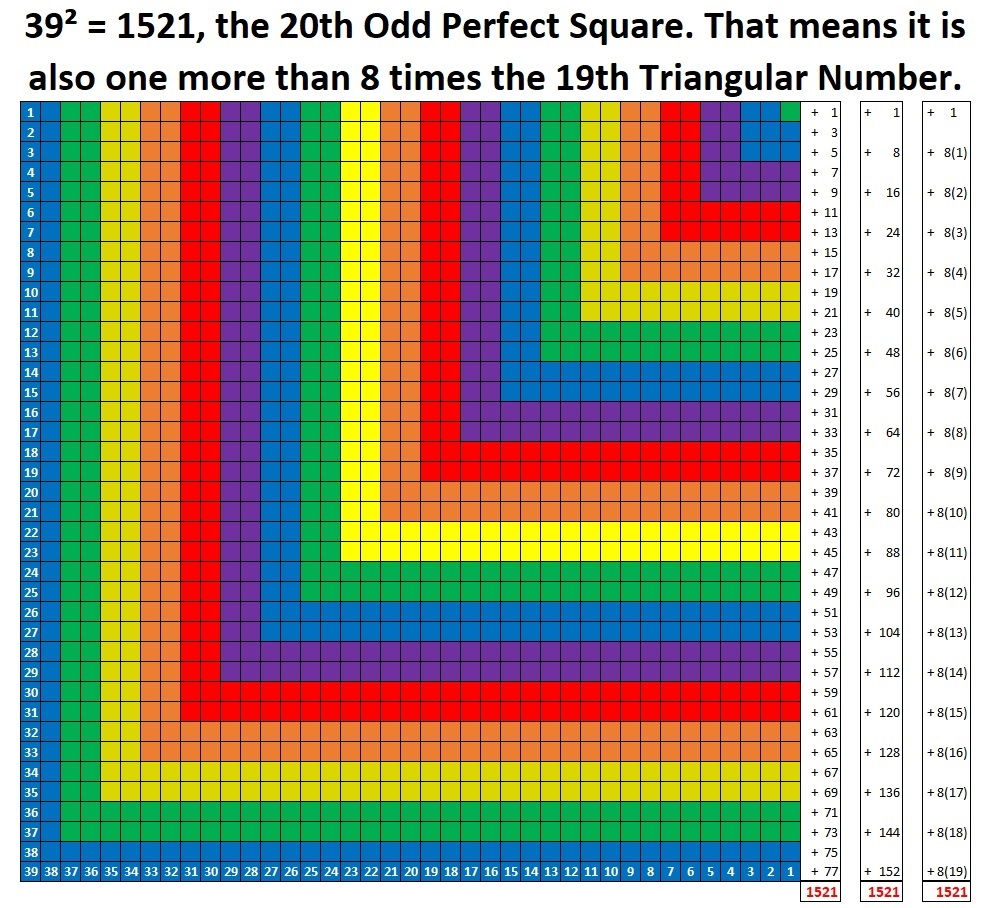# What Kind of Shape Is 1521 in?

Contents

### Today’s Puzzle:

1521 is a perfect square so if you had 1521 tiny squares, you could arrange them into a square perfectly. The following graphic has three sums on the right. The first sum shows that 1521 is the sum of the first 39 odd numbers. The last two sums prove that it is one more than eight times the 19th triangular number.Since 1521 is one more than eight times the 19th triangular number, it is also the 20th centered octagonal number. Today’s puzzle: How do we arrange 1521 tiny dots into a centered octagon?

I wanted to make a graphic of centered octagonal number 1521. I puzzled over how I could do that for several days. I decided to make it in Desmos. Since octagons are symmetrical, I knew that I could make 1/4 of it in Desmos and duplicate the other quarters in Paint. At first, I spent a lot of time typing ordered pairs in Desmos, until I realized how quickly I could make all the needed ordered pairs in Excel:

I made twenty 2-column ordered pairs in Excel. The top of each column was
(0, 0), (0, 1), (0, 2), etc . After I finished typing all of those in, I typed in the second ordered pair in each column from 2-20. I typed in the numerical value of (√2/2, (n-1) + √2/2) where n was the column number.

Then I used the drag function in Excel to complete columns 3 to 20. I dragged the first two ordered pairs in each column until I got an ordered pair where x = y. I made that last ordered pair be red.

Here is an example of how I build one column of ordered pairs:I found it easier to do a step for every column of ordered pairs before moving onto the next step. Since I wanted these points AND their inverses, I copied and pasted the non-red numbers from the x column into the y column and vice-versa.

Then I used the sort feature in Excel to get all the ordered pairs in ascending order of the x’s.

Lastly, I copied and pasted each column of ordered pairs in Desmos, and my quarter centered octagon quickly grew bigger and bigger with each paste. I found it quite enjoyable to do!

My octagon has lines and numbers on it and it wasn’t symmetrical so I needed to clean up the picture. I clicked on the wrench in Desmos, clicked on “Zoom Square” and removed all the checkmarks I saw. Then I clicked on the gear found above the ordered pairs in Desmos, clicked on the colored circles for each set of ordered pairs, and then clicked on the circle next to the word “lines,” and chose the solid line.

After I put the quarter octagon into Paint, I rotated copies of it every which way, added some words and brown lines until I got this beauty:It was so much fun to make, I encourage you to give it a try! I bet you can clearly see that 1521 is one more than eight times the 19th triangular number!

### Factors of 1521:

• 1521 is a composite number.
• Prime factorization: 1521 = 3 × 3 × 13 × 13, which can be written 1521 = 3²× 13².
• 1521 has at least one exponent greater than 1 in its prime factorization so √1521 can be simplified. Taking the factor pair from the factor pair table below with the largest square number factor, we get √1521 = (√9)(√169) = (3)(13) = 39.
• The exponents in the prime factorization are 1 and 1. Adding one to each exponent and multiplying we get (2 + 1)(2 + 1) = 3 × 3 = 9. Therefore 1521 has exactly 9 factors.
• The factors of 1521 are outlined with their factor pair partners in the graphic below.### More Facts about the Number 1521:

1521 is the sum of two squares:
36² + 15² = 1521.

1521 is the hypotenuse of two Pythagorean triples:
585-1404-1521, which is (5-12-13) times 117, and
1071-1080-1521, calculated from 36² – 15², 2(36)(15), 36² + 15².

From OEIS.org we learn that 1521 is sum of 4 distinct cubes in 3 different ways:
11³ +  5³ +  4³ +  1³ = 1521,
10³ +  8³ +  2³ +  1³ = 1521, and
9³ +  8³ +  6³ +  4³ = 1521.

We also learn that 1520 and 1521 are a Ruth-Aaron pair because they are consecutive numbers and the sum of their factors are equal to each other:
1520 = 2⁴·5·19 and 1521 = 3²·13², while
2+2+2+2+5+19 = 32=3+3+13+13.

This site uses Akismet to reduce spam. Learn how your comment data is processed.